## Hyperfine Splitting

We can think of the nucleus as a single particle with spin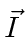. This particle is actually made up of protons and neutrons which are both spinparticles. The protons and neutrons in turn are made of spinquarks. The magnetic dipole moment due to the nuclear spin is much smaller than that of the electron because the mass appears in the denominator. The magnetic moment of the nucleus iswhereis the nuclear spin vector. Because the nucleus has internal structure, the nuclear gyromagnetic ratio is not just 2. For the proton, it is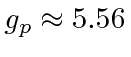. This is the nucleus of hydrogen upon which we will concentrate. Even though the neutron is neutral, the gyromagnetic ratio is about -3.83. (The quarks have gyromagnetic ratios of 2 (plus corrections) like the electron but the problem is complicated by the strong interactions which make it hard to define a quark's mass.) We can compute (to some accuracy) the gyromagnetic ratio of nuclei from that of protons and neutrons as we can compute the proton's gyromagnetic ratio from its quark constituents.

In any case, the nuclear dipole moment is about 1000 times smaller than that for e-spin or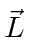. We will calculateforstates (see Condon and Shortley for more details). This is particularly important because it will break the degeneracy of the Hydrogen ground state.

To get the perturbation, we should findfrom(see Gasiorowicz page 287) then calculate the energy change in first order perturbation theory. Calculating the energy shift forstates.Now, just as in the case of the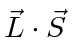, spin-orbit interaction, we will define the total angular momentumIt is in the states of definiteandthat the hyperfine perturbation will be diagonal. In essence, we are doing degenerate state perturbation theory. We could diagonalize the 4 by 4 matrix for the perturbation to solve the problem or we can use what we know to pick the right states to start with. Again like the spin orbit interaction, the total angular momentum states will be the right states because we can write the perturbation in terms of quantum numbers of those states.For the hydrogen ground state we are just adding two spinparticles so the possible values are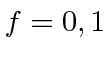.

* Example: The Hyperfine Splitting of the Hydrogen Ground State.*

The transition between the two states gives rise to EM waves with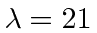cm.

Jim Branson 2013-04-22